1 回顾YOLOv1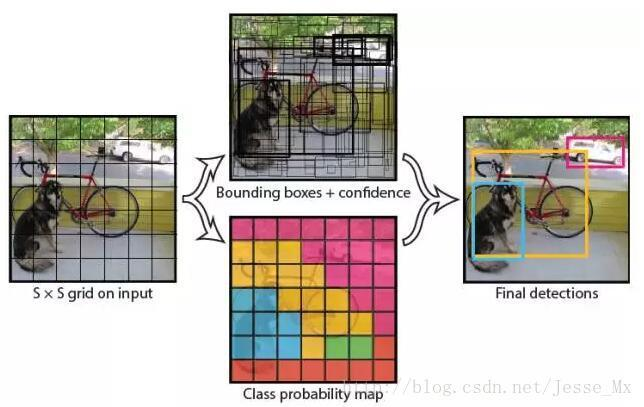1. 给个一个输入图像，首先将图像划分成 $$7\times 7$$ 的网格。

2. 对于每个网格，每个网格预测2个bouding box（每个box包含5个预测量）以及20个类别概率，总共输出 $$7\times 7\times （2\times 5+20）=1470$$ 个tensor

3. 根据上一步可以预测出 $$7 \times 7 \times 2 = 98$$ 个目标窗口，然后根据阈值去除可能性比较低的目标窗口，再由NMS去除冗余窗口即可。

YOLOv1使用了end-to-end的回归方法，没有region proposal步骤，直接回归便完成了位置和类别的判定。种种原因使得YOLOv1在目标定位上不那么精准，直接导致YOLO的检测精度并不是很高。

2 YOLOv2 的改进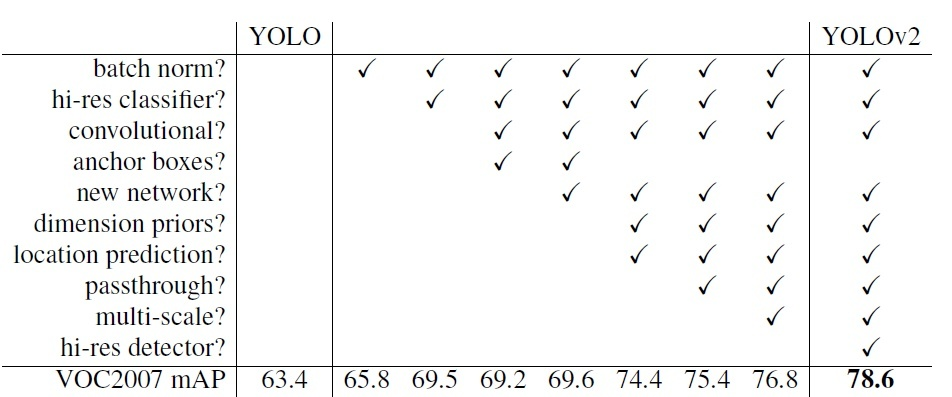2.1 Batch Normalization

CNN在训练过程中网络每层输入的分布一直在改变, 会使训练过程难度加大，但可以通过normalize每层的输入解决这个问题。新的YOLO网络在每一个卷积层后添加batch normalization，通过这一方法，mAP获得了2%的提升。batch normalization 也有助于规范化模型，可以在舍弃dropout优化后依然不会过拟合。

2.3 Convolutional With Anchor Boxes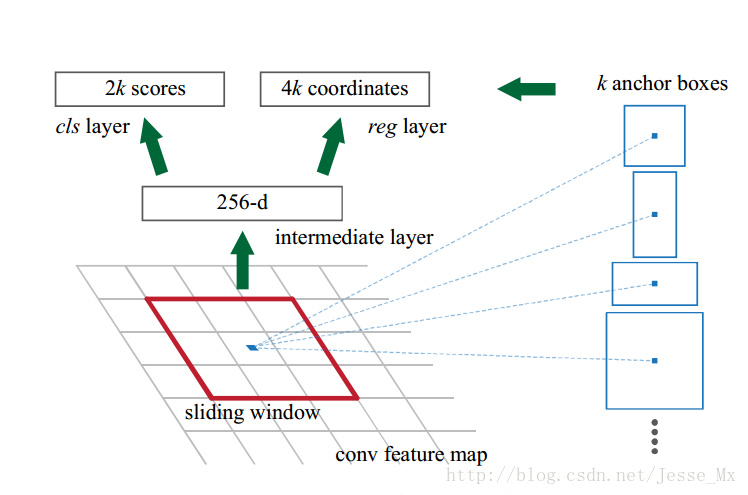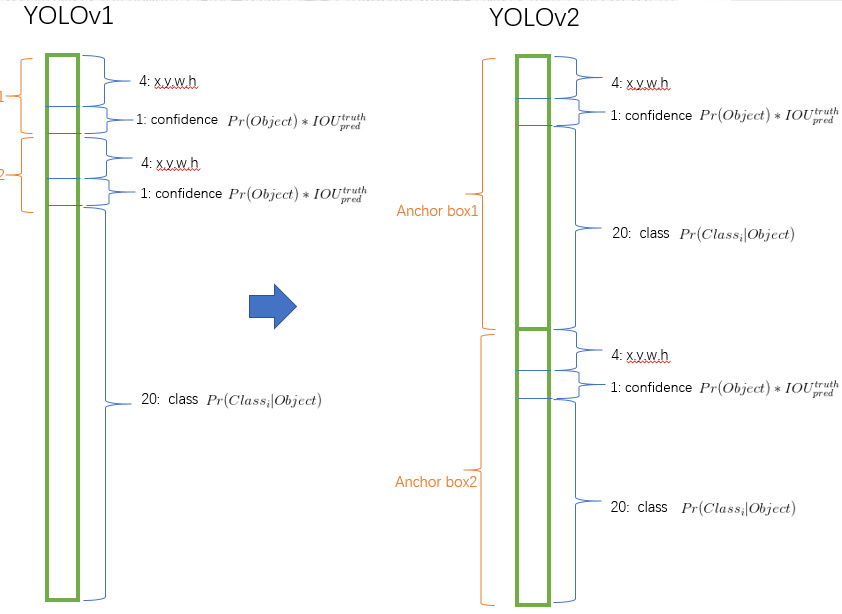2.4 Dimension Clusters（维度聚类）

YOLOv2中利用K-means聚类方法，通过对数据集中的ground truth box做聚类，找到ground truth box的统计规律，从而可以自动找到更好的boxes宽高维度。以聚类个数k为anchor boxes个数，以k个聚类中心box的宽高维度为anchor box的维度。传统的K-means聚类方法使用的是欧氏距离函数，也就意味着较大的boxes会比较小的boxes产生更多的error，聚类结果可能会偏离。为此，作者采用的评判标准是IOU得分（也就是boxes之间的交集除以并集），这样的话，error就和box的尺度无关了，聚类的距离度量采用：

$$d(box, centroid) = 1 - IOU(box, centroid)$$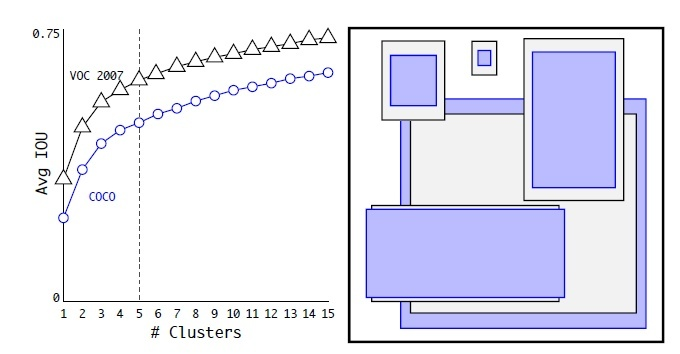2.5 Direct Location prediction

$$x = (t_x\times w_a)-x_a$$

$$y=(t_y\times h_a) - y_a$$

$$b_x = \sigma (t_x)+c_x$$

$$b_y = \sigma (t_y) + c_y$$

$$b_w = p_we^{t_w}$$

$$b_h = p_he^{t_h}$$

$$b_x = (\sigma (t_x)+c_x)/W$$

$$b_y = (\sigma (t_y) + c_y)/H$$

$$b_w = p_we^{t_w}/W$$

$$b_h = p_he^{t_h}/H$$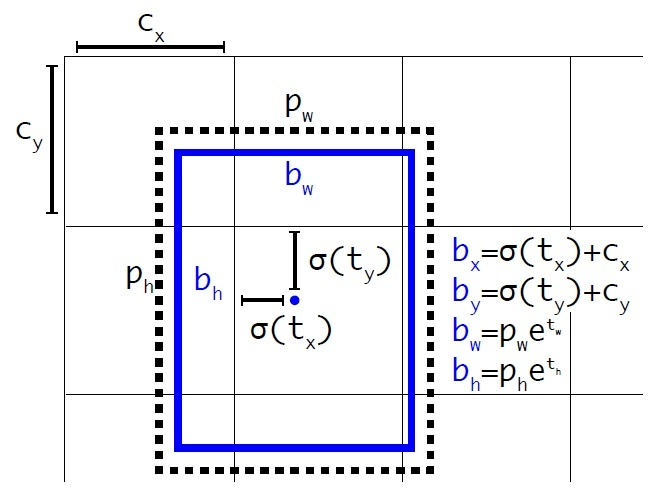2.6 New Network: Darknet-19

YOLOv2采用了一个新的基础模型（特征提取器），称为Darknet-19，包括19个卷积层和5个maxpooling层，如图4所示。Darknet-19与VGG16模型设计原则是一致的，主要采用 $$3\times3$$ 卷积，采用 $$2\times2$$ 的maxpooling层之后，特征图维度降低2倍，而同时将特征图的channles增加两倍。与NIN(Network in Network)类似，Darknet-19最终采用global avgpooling做预测，并且在 $$3\times3$$ 卷积之间使用 $$1\times1$$ 卷积来压缩特征图channles以降低模型计算量和参数。Darknet-19每个卷积层后面同样使用了batch norm层以加快收敛速度，降低模型过拟合。在ImageNet分类数据集上，Darknet-19的top-1准确度为72.9%，top-5准确度为91.2%，但是模型参数相对小一些。使用Darknet-19之后，YOLOv2的mAP值没有显著提升，但是计算量却可以减少约33%。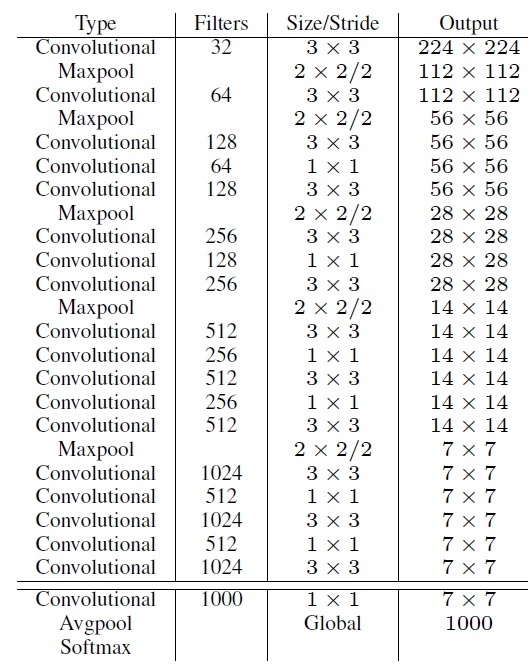2.7 Fine-Grained Features

YOLOv2的输入图片大小为 $$416\times416$$，经过5次maxpooling之后得到 $$13\times13$$ 大小的特征图，并以此特征图采用卷积做预测。 $$13\times13$$ 大小的特征图对检测大物体是足够了，但是对于小物体还需要更精细的特征图（Fine-Grained Features）。因此SSD使用了多尺度的特征图来分别检测不同大小的物体，前面更精细的特征图可以用来预测小物体。YOLOv2提出了一种passthrough层来利用更精细的特征图。YOLOv2所利用的Fine-Grained Features是 $$26\times26$$ 大小的特征图（最后一个maxpooling层的输入），对于Darknet-19模型来说就是大小为 $$26\times26\times512$$ 的特征图。passthrough层与ResNet网络的shortcut类似，以前面更高分辨率的特征图为输入，然后将其连接到后面的低分辨率特征图上。前面的特征图维度是后面的特征图的2倍，passthrough层抽取前面层的每个 $$2\times2$$ 的局部区域，然后将其转化为channel维度，对于 $$26\times26\times512$$ 的特征图，经passthrough层处理之后就变成了 $$13\times13\times2048$$的新特征图（特征图大小降低4倍，而channles增加4倍，图6为一个实例），这样就可以与后面的 $$13\times13\times1024$$ 特征图连接在一起形成 $$13\times13\times3072$$ 大小的特征图，然后在此特征图基础上卷积做预测。在YOLO的C源码中，passthrough层称为reorg layer。在TensorFlow中，可以使用tf.extract_image_patches或者tf.space_to_depth来实现passthrough层：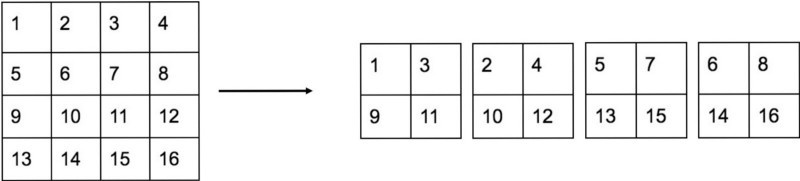2.8 Multi-Scale Training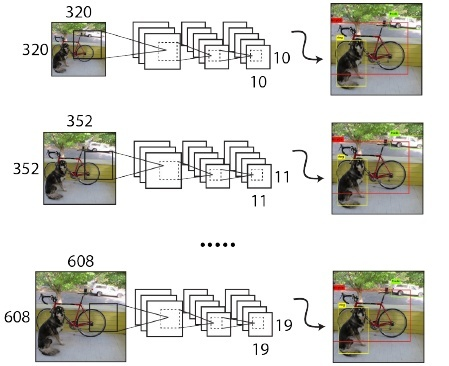2.9 总结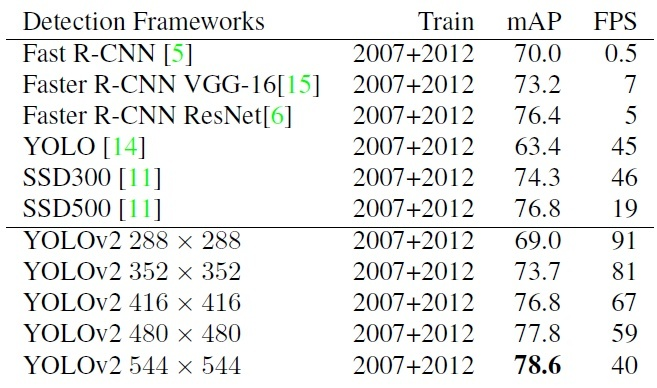3 YOLOv2 的训练

3.1 YOLOv2 的训练

YOLOv2的训练主要包括三个阶段。第一阶段就是先在ImageNet分类数据集上预训练Darknet-19，此时模型输入为 $$224\times224$$ ，共训练160个epochs。然后第二阶段将网络的输入调整为 $$448\times448$$ ，继续在ImageNet数据集上finetune分类模型，训练10个epochs，此时分类模型的top-1准确度为76.5%，而top-5准确度为93.3%。第三个阶段就是修改Darknet-19分类模型为检测模型，并在检测数据集上继续finetune网络。网络修改包括（网路结构可视化）：移除最后一个卷积层、global avgpooling层以及softmax层，并且新增了三个 $$3\times3\times2014$$ 卷积层，同时增加了一个passthrough层，最后使用 $$1\times1$$ 卷积层输出预测结果，输出的channels数为： $$\text{num_anchors}\times(5+\text{num_classes})$$ ，和训练采用的数据集有关系。由于anchors数为5，对于VOC数据集输出的channels数就是125，而对于COCO数据集则为425。这里以VOC数据集为例，最终的预测矩阵为 $$T$$（shape为 $$(\text{batch_size}, 13, 13, 125)$$ ），可以先将其reshape为 $$(\text{batch_size}, 13, 13, 5, 25)$$ ，其中 $$T[:,\ :,\ :,\ :,\ 0:4]$$ 为边界框的位置和大小 $$(t_x, t_y, t_w, t_h)$$ ， $$T[:,\ :,\ :,\ :,\ 4]$$ 为边界框的置信度，而 $$T[:,\ :,\ :,\ :,\ 5:]$$ 为类别预测值。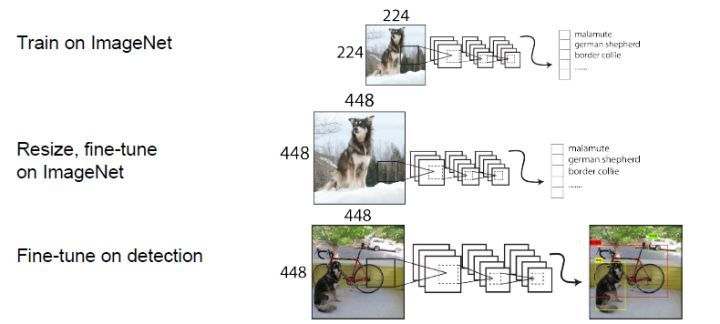YOLOv2训练的三个阶段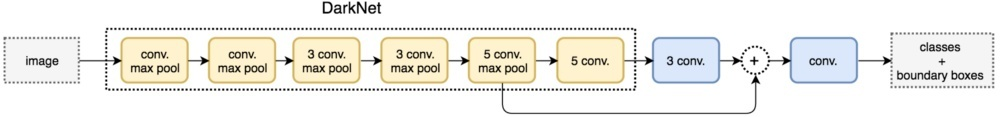YOLOv2结构示意图

YOLOv2的网络结构以及训练参数我们都知道了，但是貌似少了点东西。仔细一想，原来作者并没有给出YOLOv2的训练过程的两个最重要方面，即先验框匹配（样本选择）以及训练的损失函数，难怪Ng说YOLO论文很难懂，没有这两方面的说明我们确实不知道YOLOv2到底是怎么训练起来的。不过默认按照YOLOv1的处理方式也是可以处理，我看了YOLO在TensorFlow上的实现darkflow（见_yolov2/train.py_），发现它就是如此处理的：和YOLOv1一样，对于训练图片中的ground truth，若其中心点落在某个cell内，那么该cell内的5个先验框所对应的边界框负责预测它，具体是哪个边界框预测它，需要在训练中确定，即由那个与ground truth的IOU最大的边界框预测它，而剩余的4个边界框不与该ground truth匹配。YOLOv2同样需要假定每个cell至多含有一个grounth truth，而在实际上基本不会出现多于1个的情况。与ground truth匹配的先验框计算坐标误差、置信度误差（此时target为1）以及分类误差，而其它的边界框只计算置信度误差（此时target为0）。YOLOv2和YOLOv1的损失函数一样，为均方差函数。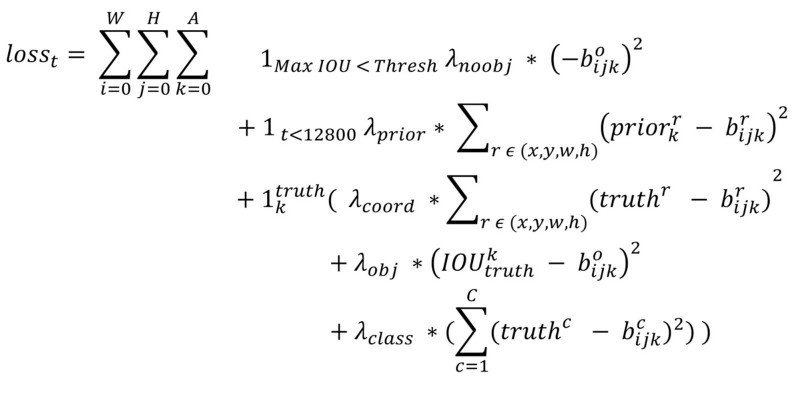3.2 YOLOv2在TensorFlow上实现

def decode(detection_feat, feat_sizes=(13, 13), num_classes=80,
anchors=None):
"""decode from the detection feature"""
H, W = feat_sizes
num_anchors = len(anchors)
detetion_results = tf.reshape(detection_feat, [-1, H * W, num_anchors,
num_classes + 5])

bbox_xy = tf.nn.sigmoid(detetion_results[:, :, :, 0:2])
bbox_wh = tf.exp(detetion_results[:, :, :, 2:4])
obj_probs = tf.nn.sigmoid(detetion_results[:, :, :, 4])
class_probs = tf.nn.softmax(detetion_results[:, :, :, 5:])

anchors = tf.constant(anchors, dtype=tf.float32)

height_ind = tf.range(H, dtype=tf.float32)
width_ind = tf.range(W, dtype=tf.float32)
x_offset, y_offset = tf.meshgrid(height_ind, width_ind)
x_offset = tf.reshape(x_offset, [1, -1, 1])
y_offset = tf.reshape(y_offset, [1, -1, 1])

# decode
bbox_x = (bbox_xy[:, :, :, 0] + x_offset) / W
bbox_y = (bbox_xy[:, :, :, 1] + y_offset) / H
bbox_w = bbox_wh[:, :, :, 0] * anchors[:, 0] / W * 0.5
bbox_h = bbox_wh[:, :, :, 1] * anchors[:, 1] / H * 0.5

bboxes = tf.stack([bbox_x - bbox_w, bbox_y - bbox_h,
bbox_x + bbox_w, bbox_y + bbox_h], axis=3)

return bboxes, obj_probs, class_probs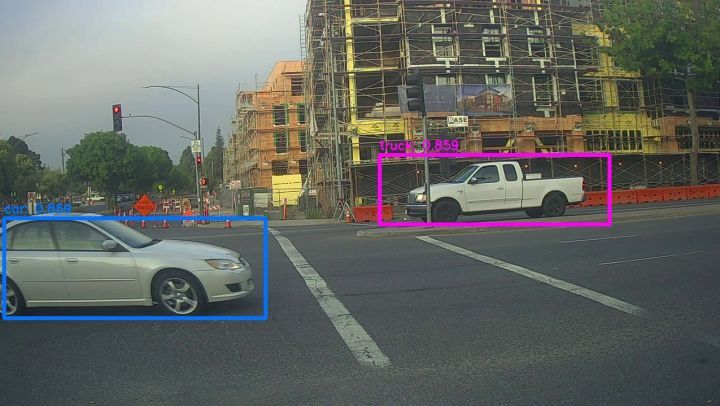4 YOLO9000

YOLO9000是在YOLOv2的基础上提出的一种可以检测超过9000个类别的模型，其主要贡献点在于提出了一种分类和检测的联合训练策略。众多周知，检测数据集的标注要比分类数据集打标签繁琐的多，所以ImageNet分类数据集比VOC等检测数据集高出几个数量级。在YOLO中，边界框的预测其实并不依赖于物体的标签，所以YOLO可以实现在分类和检测数据集上的联合训练。对于检测数据集，可以用来学习预测物体的边界框、置信度以及为物体分类，而对于分类数据集可以仅用来学习分类，但是其可以大大扩充模型所能检测的物体种类。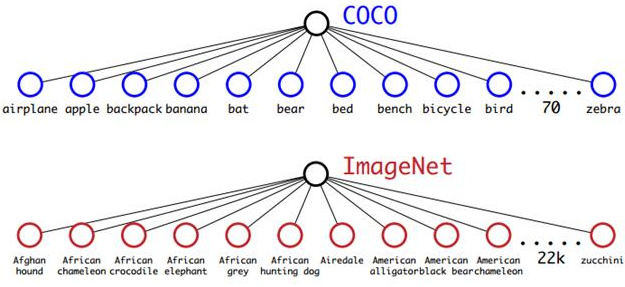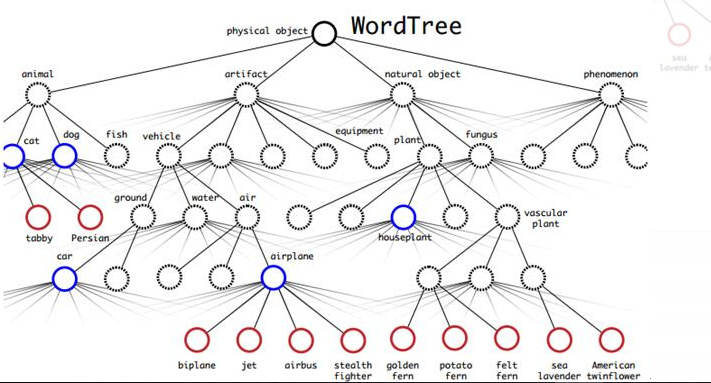WordTree中的根节点为”physical object”，每个节点的子节点都属于同一子类，可以对它们进行softmax处理。在给出某个类别的预测概率时，需要找到其所在的位置，遍历这个path，然后计算path上各个节点的概率之积。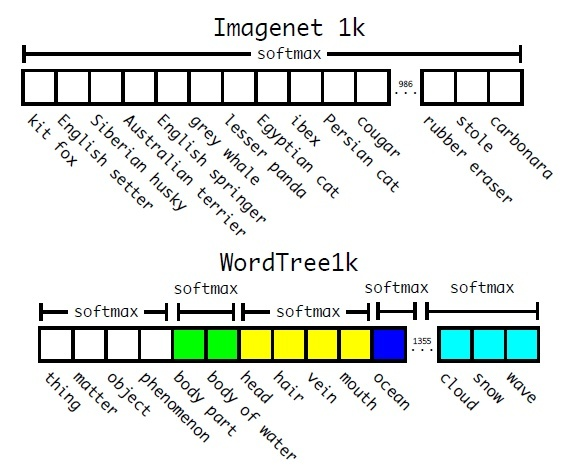ImageNet与WordTree预测的对比

5 YOLOv3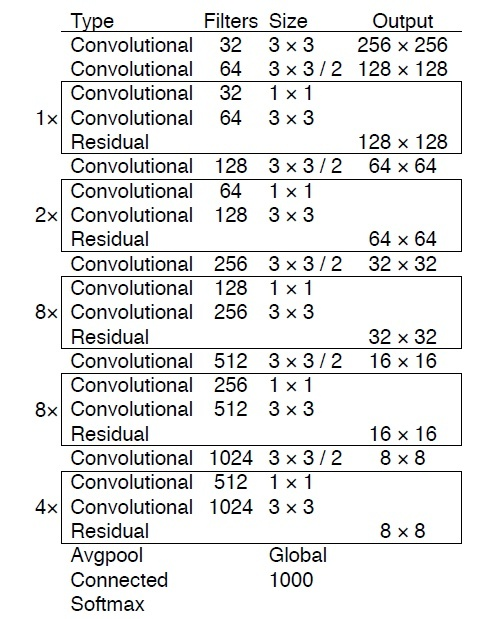YOLOv3所用的Darknet-53模型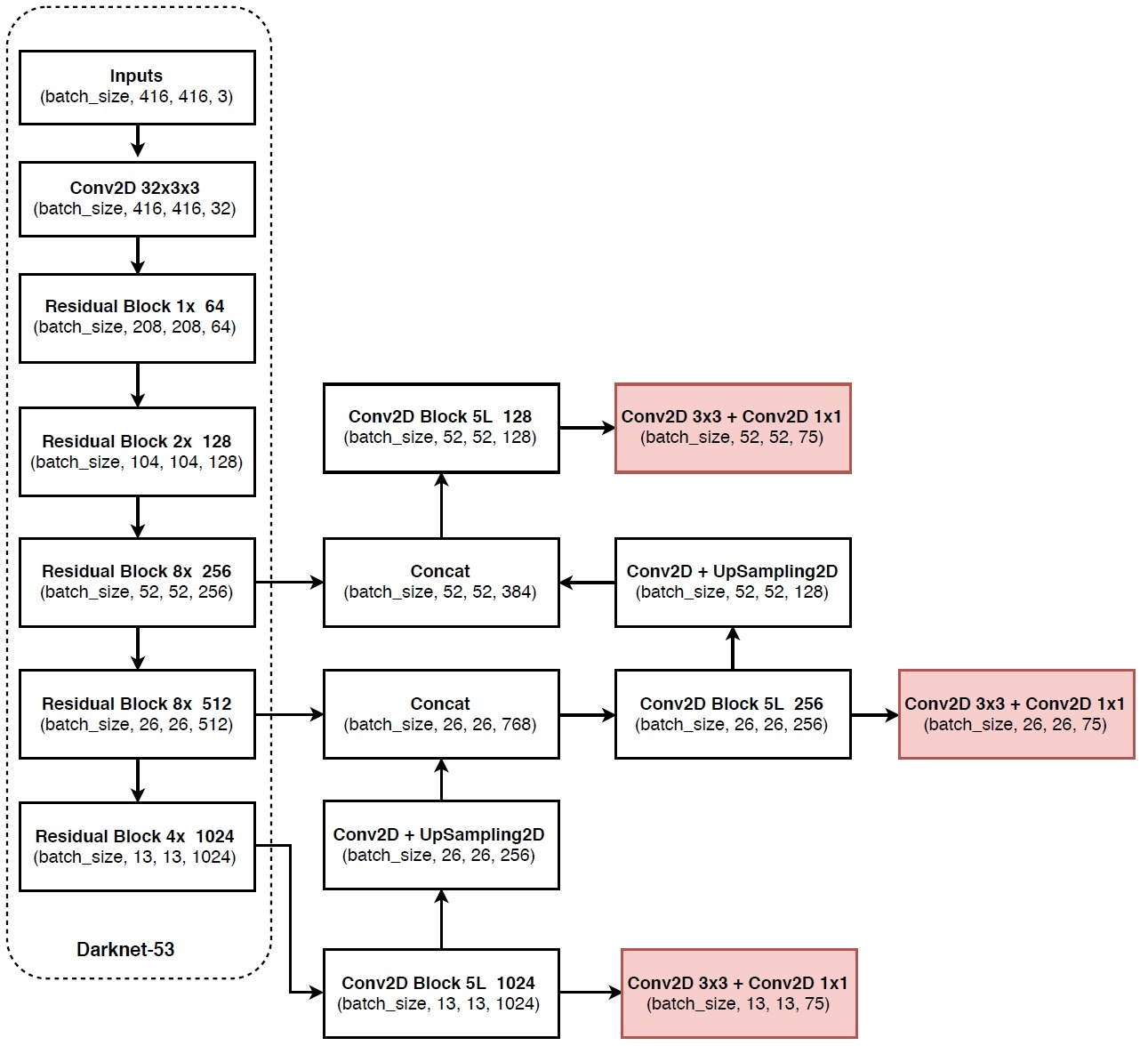YOLOv3网络结构示意图（VOC数据集）

YOLOv3与其它检测模型的对比如下图所示，可以看到在速度上YOLOv3完胜其它方法，虽然AP值并不是最好的（如果比较AP-0.5，YOLOv3优势更明显）。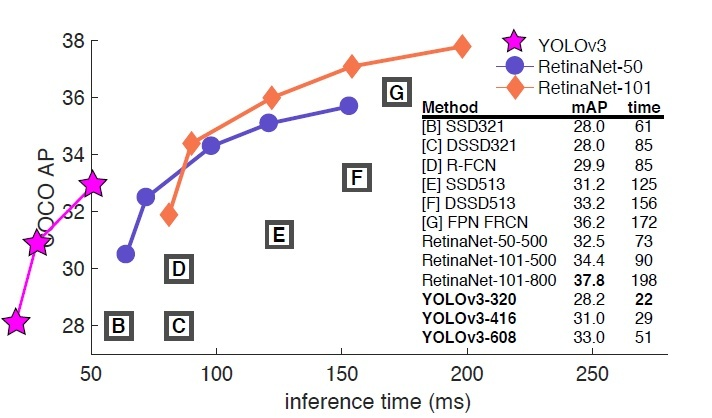YOLOv3在COCO测试集与其它检测算法对比图